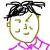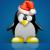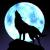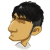## php网页地址栏信息

lv_square 发布于 2013/07/30 17:10

000```// 参数解释
// \$string： 明文 或 密文
// \$operation：DECODE表示解密,其它表示加密
// \$key： 密匙
// \$expiry：密文有效期
function authcode(\$string, \$operation = 'DECODE', \$key = '', \$expiry = 0) {
// 动态密匙长度，相同的明文会生成不同密文就是依靠动态密匙
// 加入随机密钥，可以令密文无任何规律，即便是原文和密钥完全相同，加密结果也会每次不同，增大破解难度。
// 取值越大，密文变动规律越大，密文变化 = 16 的 \$ckey_length 次方
// 当此值为 0 时，则不产生随机密钥
\$ckey_length = 4;

// 密匙
\$key = md5(\$key ? \$key : \$GLOBALS['discuz_auth_key']);

// 密匙a会参与加解密
\$keya = md5(substr(\$key, 0, 16));
// 密匙b会用来做数据完整性验证
\$keyb = md5(substr(\$key, 16, 16));
// 密匙c用于变化生成的密文
\$keyc = \$ckey_length ? (\$operation == 'DECODE' ? substr(\$string, 0, \$ckey_length): substr(md5(microtime()), -\$ckey_length)) : '';
// 参与运算的密匙
\$cryptkey = \$keya.md5(\$keya.\$keyc);
\$key_length = strlen(\$cryptkey);
// 明文，前10位用来保存时间戳，解密时验证数据有效性，10到26位用来保存\$keyb(密匙b)，解密时会通过这个密匙验证数据完整性
// 如果是解码的话，会从第\$ckey_length位开始，因为密文前\$ckey_length位保存 动态密匙，以保证解密正确
\$string = \$operation == 'DECODE' ? base64_decode(substr(\$string, \$ckey_length)) : sprintf('%010d', \$expiry ? \$expiry + time() : 0).substr(md5(\$string.\$keyb), 0, 16).\$string;
\$string_length = strlen(\$string);
\$result = '';
\$box = range(0, 255);
\$rndkey = array();
// 产生密匙簿
for(\$i = 0; \$i <= 255; \$i++) {
\$rndkey[\$i] = ord(\$cryptkey[\$i % \$key_length]);
}
// 用固定的算法，打乱密匙簿，增加随机性，好像很复杂，实际上并不会增加密文的强度
for(\$j = \$i = 0; \$i < 256; \$i++) {
\$j = (\$j + \$box[\$i] + \$rndkey[\$i]) % 256;
\$tmp = \$box[\$i];
\$box[\$i] = \$box[\$j];
\$box[\$j] = \$tmp;
}
// 核心加解密部分
for(\$a = \$j = \$i = 0; \$i < \$string_length; \$i++) {
\$a = (\$a + 1) % 256;
\$j = (\$j + \$box[\$a]) % 256;
\$tmp = \$box[\$a];
\$box[\$a] = \$box[\$j];
\$box[\$j] = \$tmp;
// 从密匙簿得出密匙进行异或，再转成字符
\$result .= chr(ord(\$string[\$i]) ^ (\$box[(\$box[\$a] + \$box[\$j]) % 256]));
}
if(\$operation == 'DECODE') {
// substr(\$result, 0, 10) == 0 验证数据有效性
// substr(\$result, 0, 10) - time() > 0 验证数据有效性
// substr(\$result, 10, 16) == substr(md5(substr(\$result, 26).\$keyb), 0, 16) 验证数据完整性
// 验证数据有效性，请看未加密明文的格式
if((substr(\$result, 0, 10) == 0 || substr(\$result, 0, 10) - time() > 0) && substr(\$result, 10, 16) == substr(md5(substr(\$result, 26).\$keyb), 0, 16)) {
return substr(\$result, 26);
} else {
return '';
}
} else {
// 把动态密匙保存在密文里，这也是为什么同样的明文，生产不同密文后能解密的原因
// 因为加密后的密文可能是一些特殊字符，复制过程可能会丢失，所以用base64编码
return \$keyc.str_replace('=', '', base64_encode(\$result));
}
}```